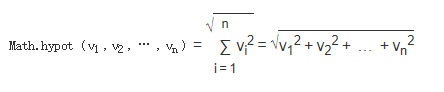# es6数值的扩展方法

更新时间：2019年03月11日 11:46:33   作者：me_zhazha我要评论

Number的方法扩展

Number.isFinite() 方法检测有限的数值（有穷的数值）

```  console.log(Number.isFinite(1)) // true
console.log(Number.isFinite(3.1415926)) // true
console.log(Number.isFinite('1')) // false
console.log(Number.isFinite(NaN)) // false
console.log(Number.isFinite('1e')) // false
console.log(isFinite('1')) // true
console.log(isFinite('1e')) // false
```

Number.isNaN() 检测一个值是不是NaN

```  console.log(Number.isNaN(1)) // false
console.log(Number.isNaN(3.1415926)) // false
console.log(Number.isNaN('1')) // false
console.log(Number.isNaN(NaN)) // true
console.log(Number.isNaN('1e')) // false
```

Number.parseInt() 隐士类型的转换

```  console.log(Number.parseInt(1)) // 1
console.log(Number.parseInt(3.1415926)) // 3
console.log(Number.parseInt(3.6415926)) // 3
console.log(Number.parseInt('1es5')) //1
console.log(Number.parseInt('asd123')) // NaN
console.log(Number.parseInt(NaN)) // NaN
```

Number.parseFloat() 类型的转换会保留浮点数

``` console.log(Number.parseFloat(1)) // 1
console.log(Number.parseFloat(3.1415926)) // 3.1415926
console.log(Number.parseFloat('1es5#')) // 1
console.log(Number.parseFloat('#asd123')) //NaN
console.log(Number.parseFloat(NaN)) // NaN
```

Number.isInteger() 检测一个值是否为整数

```console.log(Number.isInteger(1)) // true
console.log(Number.isInteger(3.1415926)) // false
console.log(Number.isInteger('123')) // false
console.log(typeof (Math.trunc('1.23'))) // number
console.log(Number.isInteger('1.23')) // false
console.log(Number.isInteger(null)) // false
console.log(Number.isInteger(3.0000000000000002)) // true
console.log(Number.parseFloat(Array)) // NaN
```

Math对象的扩展

Math.trunc() 去除浮点数的小数部分，返回整数也就相当于转换把，但是没有四舍五入

```  console.log(Math.trunc(1.8)) // 1
console.log(Math.trunc(3.1415926)) // 3
console.log(Math.trunc('3.1415926')) // 3
console.log(Math.trunc('1.23')) // 1
console.log(Math.trunc('1.23#')) // NaN
console.log(Math.trunc('#1.23')) // NaN
console.log(Math.trunc()) // NaN
console.log(Math.trunc(-0.25)) // -0```

Math.sign() 用来判断一个数到底是正数、负数、还是零。对于非数值，会先将其转换为数值

```      console.log(Math.sign(1.8))
console.log(Math.sign(3.1415926))
console.log(Math.sign('3.1415926'))
console.log(Math.sign('1.23#'))
console.log(Math.sign('#1.23'))
console.log(Math.sign(-0))
console.log(Math.sign(-2))
```

Math.cbrt() 计算立方根```console.log(Math.cbrt(4)) // 1.5874010519681996
console.log(Math.cbrt('4')) // 1.5874010519681996
console.log(Math.cbrt('2q')) // NaN
console.log(Math.cbrt('q2')) // NaN
console.log(Math.cbrt('16')) // 2.5198420997897464```

Math.hypot() 返回参数内平方的和，可以任意多个参数

```console.log(Math.hypot(4, 4)) // 5.656854249492381
console.log(Math.hypot('4', 4, 3)) // 6.4031242374328485
console.log(Math.hypot('2q', 4)) // NaN
console.log(Math.hypot('q2', 4)) // NaN
console.log(Math.hypot('16', 4)) // 16.492422502470642```

Math.log1p() 返回1+参数的自然数，如果小于-1就返回NaN

```  console.log(Math.log1p(1)) // 0.6931471805599453    也相当于0
console.log(Math.log1p(-1)) //-Infinity
console.log(Math.log1p(-2)) // NaN
console.log(Math.log1p('-1')) // -Infinity
console.log(Math.log1p('-2q')) // NaN```

Math.log10() 返回以10为底x的对数，小于0返回NaN

```console.log(Math.log10(10)) // 1
console.log(Math.log10(5)) // 0.6989700043360189
console.log(Math.log10(20)) // 1.3010299956639813
console.log(Math.log10(2000)) // 3.3010299956639813```

Math.log2() 返回以2为底x的对数，小于0返回NaN

```console.log(Math.log10(10)) // 3.321928094887362
console.log(Math.log10(5)) // 2.321928094887362
console.log(Math.log10(20)) // 4.321928094887363
console.log(Math.log10(2000)) // 10.965784284662087
console.log(Math.log2('2000')) // 10.965784284662087```